# Histogram in R using ggplot2

• Difficulty Level : Easy
• Last Updated : 25 Feb, 2021

ggplot2 is an R Package that is dedicated to Data visualization. ggplot2 Package  Improve the quality and the beauty (aesthetics ) of the graph. By Using ggplot2 we can make almost every kind of graph In RStudio

A histogram is an approximate representation of the distribution of numerical data. In a histogram, each bar groups numbers into ranges. Taller bars show that more data falls in that range. A histogram displays the shape and spread of continuous sample data.

Histograms roughly give us an idea about the probability distribution of a given variable by depicting the frequencies of observations occurring in certain ranges of values. Basically, Histograms are used to show distributions of a given variable while bar charts are used to compare variables. Histograms plot quantitative data with ranges of the data grouped into the intervals while bar charts plot categorical data.

geom_histogram() function is an in-built function of ggplot2 module.

Approach

• Import module
• Create dataframe
• Create histogram using function
• Display plot

Example 1:

## R

 `set.seed``(123) ` ` `  `# In the above line,123 is set as the  ` `# random number value ` `# The main point of using the seed is to ` `# be able to reproduce a particular sequence  ` `# of 'random' numbers. and sed(n) reproduces ` `# random numbers results by seed ` `df <- ``data.frame``( ` `   ``gender=``factor``(``rep``(``c``( ` `     ``"Average Female income "``, ``"Average Male incmome"``), each=20000)), ` `   ``Average_income=``round``(``c``(``rnorm``(20000, mean=15500, sd=500),  ` `                          ``rnorm``(20000, mean=17500, sd=600)))    ` `)   ` `head``(df) ` ` `  `# if already installed ggplot2 then use library(ggplot2) ` `library``(ggplot2) ` ` `  `# Basic histogram ` `ggplot``(df, ``aes``(x=Average_income)) + ``geom_histogram``() ` ` `  `# Change the width of bins ` `ggplot``(df, ``aes``(x=Average_income)) +     ` ` `  `   ``geom_histogram``(binwidth=1) ` ` `  `# Change colors ` `p<-``ggplot``(df, ``aes``(x=Average_income)) +    ` ` `  `   ``geom_histogram``(color=``"white"``, fill=``"red"``) ` `p`

Output :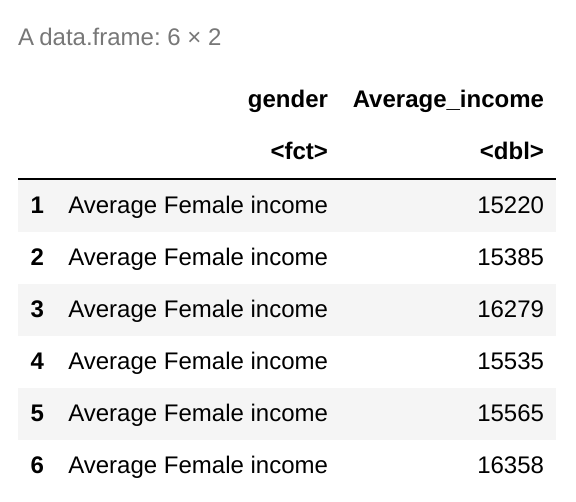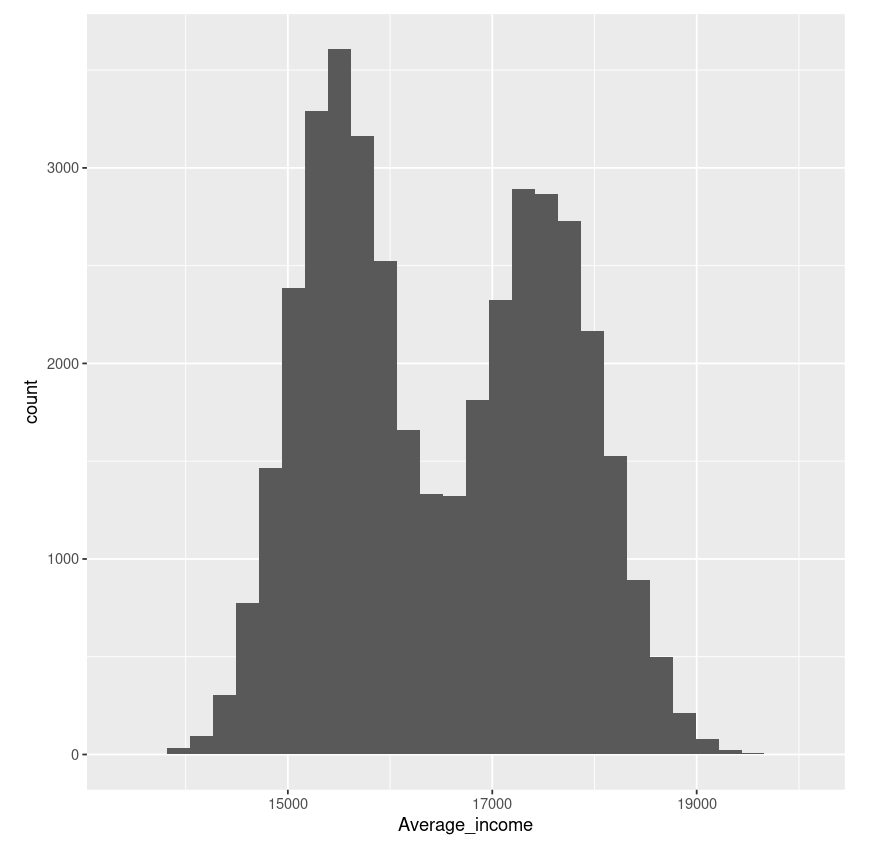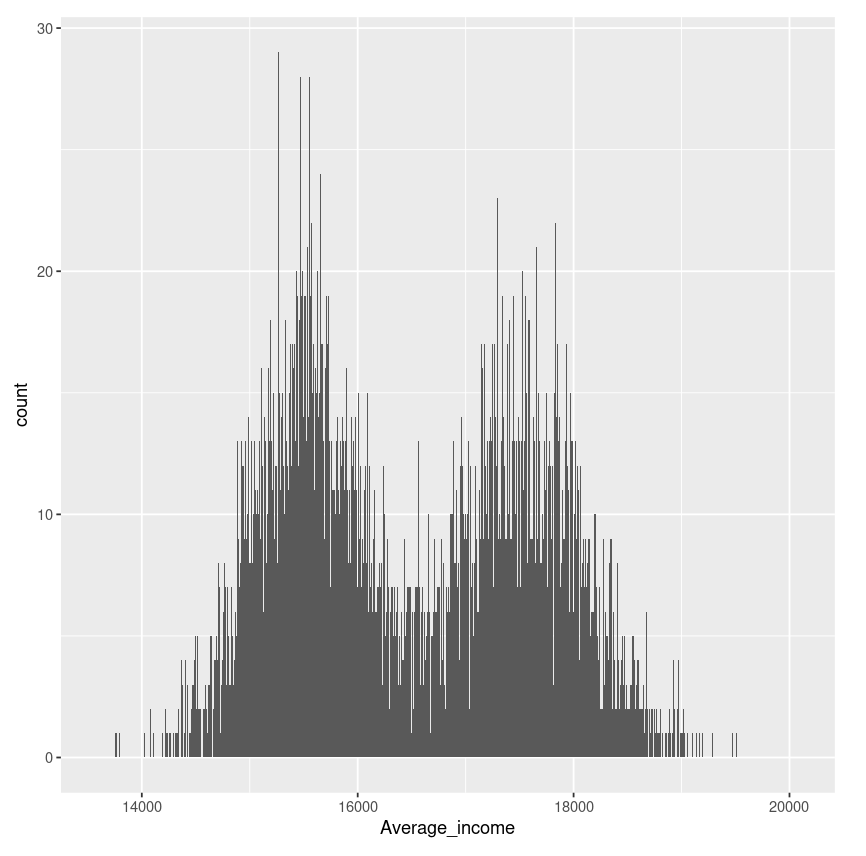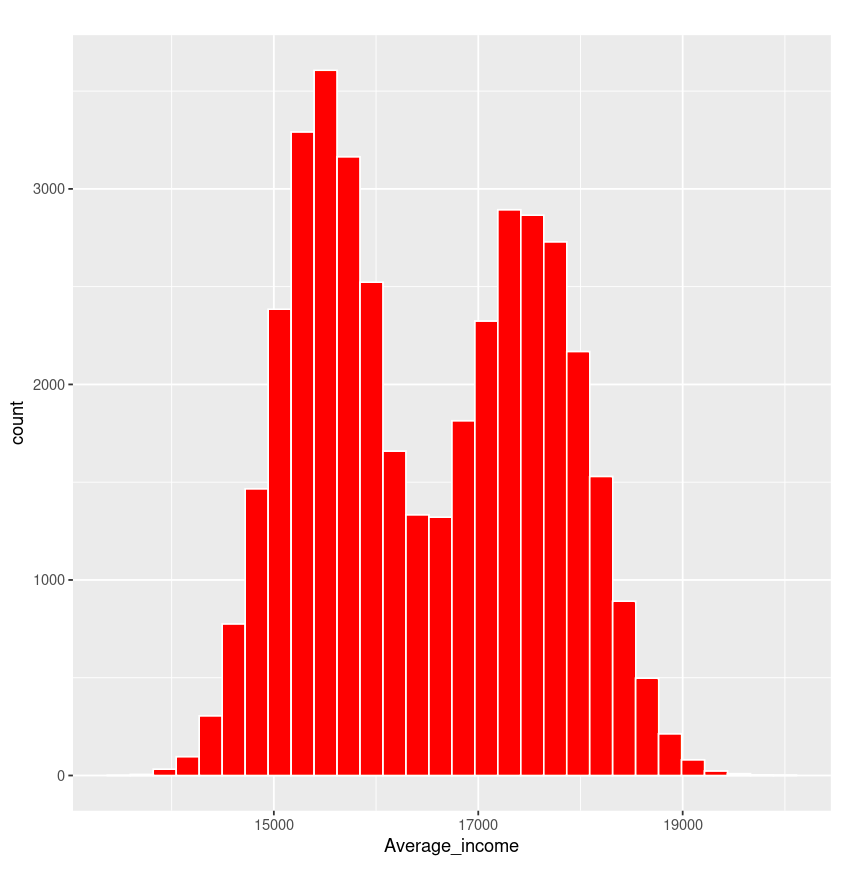Example 2:

## R

 `plot_hist <- ``ggplot``(airquality, ``aes``(x = Ozone)) + ` ` `  `   ``# binwidth help to change the thickness (Width) of the bar  ` `   ``geom_histogram``(``aes``(fill = ..count..), binwidth = 10)+ ` ` `  `   ``# name = "Mean ozone(03) in ppm parts per million " ` `   ``# name is used to give name to axis   ` `   ``scale_x_continuous``(name = ``"Mean ozone(03) in ppm parts per million "``, ` `                      ``breaks = ``seq``(0, 200, 25), ` `                      ``limits=``c``(0, 200)) + ` `   ``scale_y_continuous``(name = ``"Count"``) + ` ` `  `   ``# ggtitle is used to give name to a chart ` `   ``ggtitle``(``"Frequency of mean ozone(03)"``) + ` `   ``scale_fill_gradient``(``"Count"``, low = ``"green"``, high = ``"red"``) ` ` `  `plot_hist`

Output :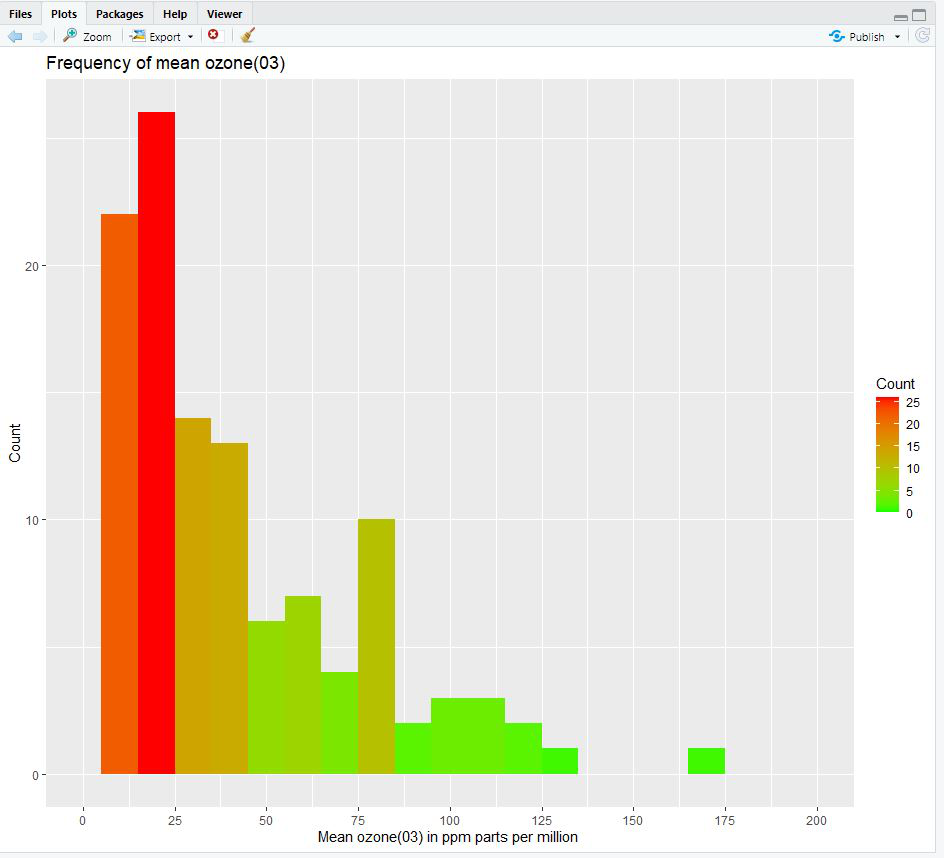My Personal Notes arrow_drop_up
Recommended Articles
Page :